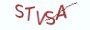Python设计模式

# 2.2.3 源码实现

```# __author__ = 薯条老师，微信公众号：薯条编程

"""

"""

"""
(1) 定义一个全局的计数器对象Counter，以饿汉模式中的单例来实现
"""

class Counter:
__instance = None
"""
(2) 重写__new__方法来实现饿汉模式的单例
"""

def __new__(cls):
if not cls.__instance:
cls.__instance = super().__new__(cls)
return cls.__instance

def __init__(self):
self._count = 0 if "_count" not in self.__dict__ else self.__dict__["_count"]

@property
def count(self):
return self._count

@count.setter
def count(self, value):
self._count += value

if __name__ == "__main__":
counter = Counter()
counter.count = 1
counter = Counter()
counter.count = 1
# 输出的结果为2，说明为同一个对象
print(counter.count)```

```# __author__ = 薯条老师，微信公众号：薯条编程

"""

"""

"""
(1) 定义一个全局的计数器对象Counter，以懒汉模式中的单例来实现
"""

class Counter:
__instance = None
"""
(2) 通过类方法来实现懒汉模式中的单例
"""
@classmethod
def get_instance(cls):
if not cls.__instance:
cls.__instance = cls()
return cls.__instance

def __init__(self):
self.__count = 0

@property
def count(self):
return self.__count

@count.setter
def count(self, value):
self.__count += value

if __name__ == "__main__":
counter = Counter.get_instance()
counter.count = 1
counter = Counter.get_instance()
counter.count = 1
print(counter.count)```

# 2.2.4 最具实力的小班培训

`(1) Python后端工程师高薪就业班，月薪11K-18K，免费领取课程大纲(2) Python爬虫工程师高薪就业班，年薪十五万，免费领取课程大纲(3) Java后端开发工程师高薪就业班，月薪11K-20K, 免费领取课程大纲(4) Python大数据分析，量化投资就业班，月薪12K-25K,免费领取课程大纲`## 注册账号